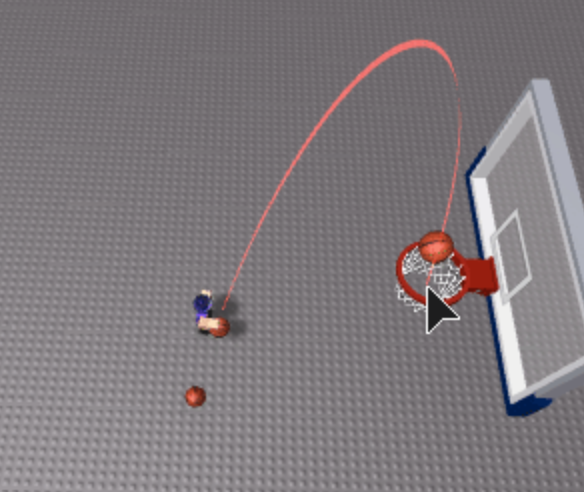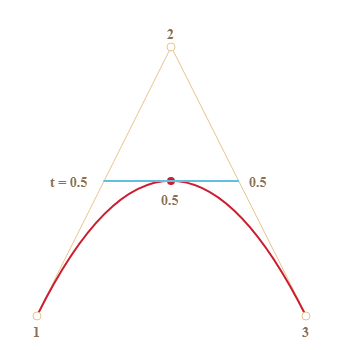# Using beams to show a projectiles predicted path

Hello

So I’m looking to do something like this to help players aim their projectilesI was reading over Egomoose’s tutorial, and realized its contingent on me knowing where the projectile will end up. However in my use case I am firing at a projectile with a velocity, and then using SUVAT calculations to move it along a path.

So I think the best way to use beams to show the projectile path is to guess where the ball is gonna end up. My current guess is just to calculate where the projectile will be in say, 10 seconds, and treat that as the end point, but maybe there is a better way to do it?

1 Like

Yes, that is probably the best way. Although I don’t know what you are doing, for collidable projectiles, I would raycast along the projectile’s path before the projectile actually starts its path and store that path, similar to pathfinding.

try using the bezier curve1 Like

Well if your using SUVAT (and RUVAT for vectors), you can calculate the exact amount of time elapsed as well as maximum height etc, as well as when it hits the ground (final velocity will be 0). Technically if you can split this entire thing into components x, y, z and work out where the projectile will end up but this is not going to be nice maths.

If you calculate where the projectile is in 10 seconds, you don’t consider if the ball has rolled since or if its hit something since. I’m not sure, but you might be able to use body movers for the speed and then handle the height yourself. This is just because the speed of the ball is unlikely to slow down that much but gravity will pull the ball down.

Its hard to explain though.